# Truncated icosidodecahedron

Truncated icosidodecahedron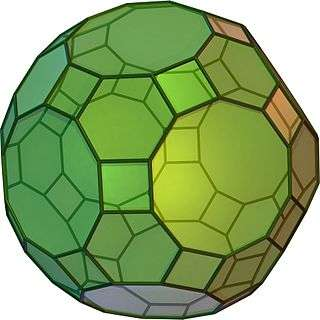TypeArchimedean solid
Uniform polyhedron
ElementsF = 62, E = 180, V = 120 (χ = 2)
Faces by sides30{4}+20{6}+12{10}
Schläfli symbolstr{5,3} ort0,1,2{5,3}
Wythoff symbol2 3 5 |
Coxeter diagramSymmetry groupIh, H3, [5,3], (*532), order 120
Rotation groupI, [5,3]+, (532), order 60
Dihedral Angle6-10: 142.62°
4-10: 148.28°
4-6: 159.095°
ReferencesU28, C31, W16
PropertiesSemiregular convex zonohedron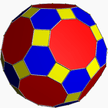Colored faces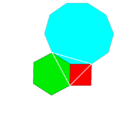4.6.10
(Vertex figure)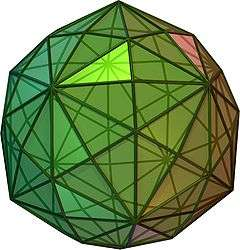Disdyakis triacontahedron
(dual polyhedron)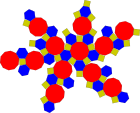Net

In geometry, the truncated icosidodecahedron is an Archimedean solid, one of thirteen convex isogonal nonprismatic solids constructed by two or more types of regular polygon faces.

It has 30 square faces, 20 regular hexagonal faces, 12 regular decagonal faces, 120 vertices and 180 edges – more than any other convex nonprismatic uniform polyhedron. Since each of its faces has point symmetry (equivalently, 180° rotational symmetry), the truncated icosidodecahedron is a zonohedron.

## Other names

Alternate interchangeable names include:

The name truncated icosidodecahedron, originally given by Johannes Kepler, is somewhat misleading. If one truncates an icosidodecahedron by cutting the corners off, one does not get this uniform figure: instead of squares the truncation has golden rectangles. However, the resulting figure is topologically equivalent to this and can always be deformed until the faces are regular.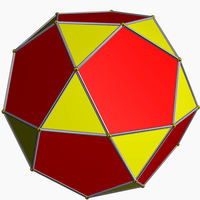Icosidodecahedron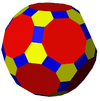A literal geometric truncation of the icosidodecahedron produces rectangular faces rather than squares.

The alternative name great rhombicosidodecahedron (as well as rhombitruncated icosidodecahedron) refers to the fact that the 30 square faces lie in the same planes as the 30 faces of the rhombic triacontahedron which is dual to the icosidodecahedron. Compare to small rhombicosidodecahedron.

One unfortunate point of confusion is that there is a nonconvex uniform polyhedron of the same name. See nonconvex great rhombicosidodecahedron.

## Area and volume

The surface area A and the volume V of the truncated icosidodecahedron of edge length a are:If a set of all 13 Archimedean solids were constructed with all edge lengths equal, the truncated icosidodecahedron would be the largest.

## Cartesian coordinates

Cartesian coordinates for the vertices of a truncated icosidodecahedron with edge length 2φ  2, centered at the origin, are all the even permutations of:

1/φ, ±1/φ, ±(3 + φ)),
2/φ, ±φ, ±(1 + 2φ)),
1/φ, ±φ2, ±(−1 + 3φ)),
(±(2φ  1), ±2, ±(2 + φ)) and
(±φ, ±3, ±2φ),

where φ = 1 + 5/2 is the golden ratio.

## Orthogonal projections

The truncated icosidodecahedron has seven special orthogonal projections, centered on a vertex, on three types of edges, and three types of faces: square, hexagonal and decagonal. The last two correspond to the A2 and H2 Coxeter planes.

Orthogonal projections
Centered by Vertex Edge
4-6
Edge
4-10
Edge
6-10
Face
square
Face
hexagon
Face
decagon
Image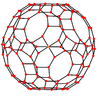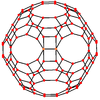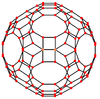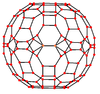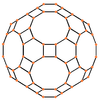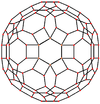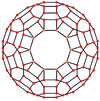Projective
symmetry
+      
Dual
image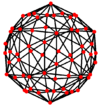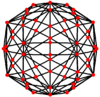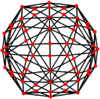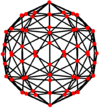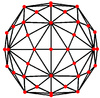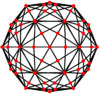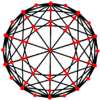## Spherical tilings and Schlegel diagrams

The truncated icosidodecahedron can also be represented as a spherical tiling, and projected onto the plane via a stereographic projection. This projection is conformal, preserving angles but not areas or lengths. Straight lines on the sphere are projected as circular arcs on the plane.

Schlegel diagrams are similar, with a perspective projection and straight edges.

Orthographic projection Stereographic projections
Decagon-centered Hexagon-centered Square-centered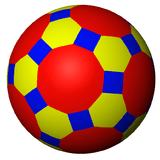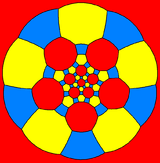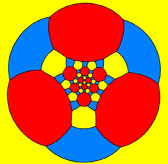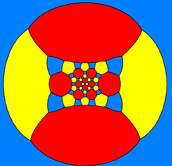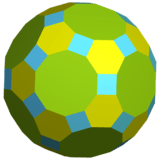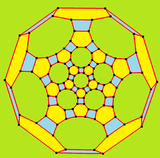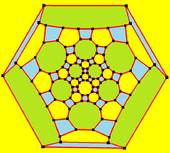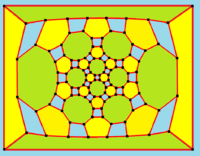## Geometric variations

Within Icosahedral symmetry there are unlimited geometric variations of the truncated icosidodecahedron with isogonal faces. The truncated dodecahedron, rhombicosidodecahedron, and truncated icosahedron as degenerate limiting cases.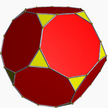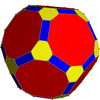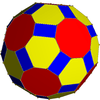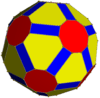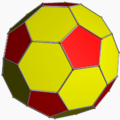## Truncated icosidodecahedral graph

Truncated icosidodecahedral graph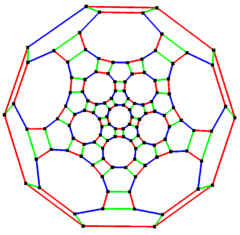5-fold symmetry
Vertices 120
Edges 180
Diameter 15
Girth 4
Automorphisms 120 (A5×2)
Chromatic number 2
Properties Cubic, Hamiltonian, regular, zero-symmetric

In the mathematical field of graph theory, a truncated icosidodecahedral graph (or great rhombicosidodecahedral graph) is the graph of vertices and edges of the truncated icosidodecahedron, one of the Archimedean solids. It has 120 vertices and 180 edges, and is a zero-symmetric and cubic Archimedean graph.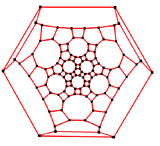3-fold symmetry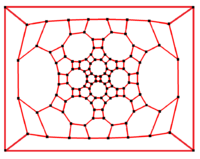2-fold symmetry

This polyhedron can be considered a member of a sequence of uniform patterns with vertex figure (4.6.2p) and Coxeter-Dynkin diagram. For p < 6, the members of the sequence are omnitruncated polyhedra (zonohedrons), shown below as spherical tilings. For p > 6, they are tilings of the hyperbolic plane, starting with the truncated triheptagonal tiling.

1. Wenninger, (Model 16, p. 30)
2. Williamson (Section 3-9, p. 94)
3. Cromwell (p. 82)
4. Read, R. C.; Wilson, R. J. (1998), An Atlas of Graphs, Oxford University Press, p. 269

• *